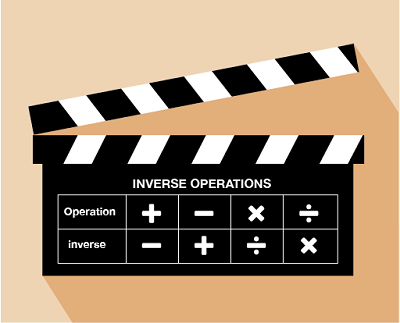Everyday maths 2

Start this free course now. Just create an account and sign in. Enrol and complete the course for a free statement of participation or digital badge if available.

Free course

# 9 Checking your answersFigure 23 Inverse operations

An inverse operation is an opposite operation. In a sense, it ‘undoes’ the operation that has just been performed. Let’s look at two simple examples to begin with.

## Example: Check your working 1

6 + 10 = 16

### Method

Since you have done an addition sum, the inverse operation is subtraction. To check this calculation, you can either do:

16 − 10 = 6

or

16 − 6 = 10

You will notice here that the same 3 numbers (6, 10 and 16) have been used in all the calculations.

## Example: Check your working 2

5 × 3 = 15

### Method

This time, since you have done a multiplication sum, the inverse operation is division. To check this calculation, you can either do:

15 ÷ 5 = 3

or

15 ÷ 3 = 5

Again, you will notice that the same 3 numbers (3, 5 and 15) have been used in all the calculations.

If you have done a more complicated calculation, involving more than one step, you simply ‘undo’ each step.

## Example: Check your working 3

A coat costing £40 has a discount of 15%. How much do you pay?

### Method

Firstly, we find out 15% of £40:

40 ÷ 100 × 15 = £6 discount

£40 − £6 = £34 to pay

To check this calculation, firstly you would check the subtraction sum by doing the addition:

£34 + £6 = £40

To check the percentage calculation, you then do:

£6 ÷ 15 × 100 = £40

You have now completed the number section of the course. Before moving on to the next session, ‘Units of measure’, complete the quiz on the following page to check your knowledge and understanding.

## Summary

In this section you have:

• learned that each of the four operations has an inverse operation (its opposite) and that these can be used to check your answers
• seen examples and practised checking answers using the inverse operation.
FSM_2

### Take your learning further

Making the decision to study can be a big step, which is why you'll want a trusted University. The Open University has 50 years’ experience delivering flexible learning and 170,000 students are studying with us right now. Take a look at all Open University courses.

If you are new to University-level study, we offer two introductory routes to our qualifications. You could either choose to start with an Access module, or a module which allows you to count your previous learning towards an Open University qualification. Read our guide on Where to take your learning next for more information.

Not ready for formal University study? Then browse over 1000 free courses on OpenLearn and sign up to our newsletter to hear about new free courses as they are released.

Every year, thousands of students decide to study with The Open University. With over 120 qualifications, we’ve got the right course for you.

Request an Open University prospectus371